## Background information

When you create a table, you can define one or more columns as the sort key. After data is written to the table, you can sort the table data by sort key.

Sorting accelerates range-restricted queries. The minimum and maximum values for each column are stored in a database. If a query restricts the range in the WHERE clause, the query processor of AnalyticDB for PostgreSQL can use the minimum and maximum values to skip blocks out of the specific range during table scans.

For example, assume that a table stores seven years of data sorted by date, and a query specifies a date range of one month. In this case, only 1/(7 × 12) of the table data needs to be scanned, and 98.8% of the disk blocks can be eliminated from the scan. If the data is not sorted by date, all disk blocks may be scanned.

AnalyticDB for PostgreSQL supports two sorting methods:

• Compound sorting: applies to scenarios where the confining condition (constraint in the WHERE clause) is a prefix subset of the sort key or consists of all columns of the sort key. This sorting method is more useful for scenarios where the query condition includes the primary column of the confining condition.
• Interleaved sorting: gives an equal weight to each column in the sort key. This sorting method is more useful for scenarios where the query condition includes a subset of the confining condition.

## Select a sort key

If you always need to run SQL queries that contain one or more column values or that are range-restricted, such as date columns, you can use those columns as sort keys. This accelerates the preceding SQL queries by sorting data based on rough set indexes. We recommend that you use compound sorting in general cases.

If you seldom need to run SQL queries that contain specific columns, you can use interleaved sorting to accelerate queries. An interleaved sort key can use up to eight columns. In general cases, interleaved sorting takes longer than compound sorting. This is because interleaved sorting needs to perform extra analysis on the data.

If you frequently use a fixed column as the JOIN condition, you can specify the JOIN column as both the distribution key and the sort key. This allows you to choose a faster merge join instead of a hash join. The sort phase of the sort-merge join can be bypassed because the data is already sorted based on the join key.

## Performance comparison between compound sorting and interleaved sorting

In this section, two tables that contain the same data are separately sorted by using compound sorting and interleaved sorting. The results of queries on the tables show that the two sorting methods provide different performance levels in different scenarios. Two tables with the same four columns (id, num1, num2, and value) are used in this example. (id,num1,num2) is specified as the sort key. Each table contains a total of 10 million rows. The tables used in this example are not particularly large tables for AnalyticDB for PostgreSQL, but the performance difference between compound sorting and interleaved sorting can be seen with the tables. The performance difference is more significant in large tables.

1. Create two tables and set the same sort key for them.
``````CREATE TABLE test(id int, num1 int, num2 int, value varchar)
with(APPENDONLY=TRUE, ORIENTATION=column)
DISTRIBUTED BY(id)
SORTKEY(id, num1, num2);

CREATE TABLE test_multi(id int, num1 int, num2 int, value varchar)
with(APPENDONLY=TRUE, ORIENTATION=column)
DISTRIBUTED BY(id)
SORTKEY(id, num1, num2);``````
2. Write test data to the tables.
``````INSERT INTO test(id, num1, num2, value) select g,
(random()*10000000)::int,
(random()*10000000)::int,
(array['foo', 'bar', 'baz', 'quux', 'boy', 'girl', 'mouse', 'chlid', 'phone'])[floor(random() * 10 +1)]
FROM generate_series(1, 10000000) as g;

INSERT INTO test_multi SELECT * FROM test;

count
----------
10000000
(1 row)

count
----------
10000000
(1 row)``````
3. Separately sort the two tables by using compound sorting and interleaved sorting.
``````SORT test;
MULTISORT test_multi;``````
4. Compare the point query performance.
• The query is filtered based on the primary column.
``````-- Q1 is filtered based on the primary column.
select * from test where id = 100000;
select * from test_multi where id = 100000;``````
• The query is filtered based on the second column.
``````-- Q2 is filtered based on the second column.
select * from test where num1 = 8766963;
select * from test_multi where num1 = 8766963;``````
• The query is filtered based on the second and third columns.
``````-- Q3 is filtered based on the second and third columns.
select * from test where num1 = 100000 and num2=2904114;
select * from test_multi where num1 = 100000 and num2=2904114;``````

Performance comparison results

Sorting method Q1 Q2 Q3
Compound sorting 0.026s 3.95s 4.21s
Interleaved sorting 0.55s 0.42s 0.071s
5. Compare the range query performance.
• The query is filtered based on the primary column.
``````-- Q1 is filtered based on the primary column.
select count(*) from test where id>5000 and id < 100000;
select count(*) from test_multi where id>5000 and id < 100000;``````
• The query is filtered based on the second column.
``````-- Q2 is filtered based on the second column.
select count(*) from test where num1 >5000 and num1 <100000;
select count(*) from test_multi where num1 >5000 and num1 <100000;``````
• The query is filtered based on the second and third columns.
``````-- Q3 is filtered based on the second and third columns.
select count(*) from test where num1 >5000 and num1 <100000; and num2 < 100000;
select count(*) from test_multi where num1 >5000 and num1 <100000 and num2 < 100000;``````

Performance comparison results

Sorting method Q1 Q2 Q3
Compound sorting 0.07s 3.35s 3.64s
Interleaved sorting 0.44s 0.28s 0.047s

Test conclusion

• Q1 uses the primary column of the sort key to filter data. In this case, compound sorting has a shorter query response time than interleaved sorting.
• Q2 uses a non-primary key column of the sort key to filter data. In this case, compound sorting is ineffective and interleaved sorting has significantly better query performance.
• Q3 uses non-primary key columns of the sort key to filter data. In this case, interleaved sorting is faster and more effective than compound sorting. The more columns of interleaved sort keys used, the better the performance of the interleaved sorting query.

## Sorting acceleration

After you execute a `SORT <tablename>` statement, the system sorts the data of the specified table. Then, AnalyticDB for PostgreSQL pushes operators such as SORT down to the storage layer so that queries are accelerated based on the physical order of data. When your underlying data is ordered, your queries can be accelerated. This feature can accelerate queries that contain SORT, AGG, or JOIN operators based on sort keys.

Note
• The sorting acceleration feature requires all of your data to be ordered. After you write data, you must execute the `SORT <tablename>` statement again to sort data.
• By default, the sorting acceleration feature is enabled.

In this example, a test table named far is used to compare the query time before and after sorting acceleration.

1. Execute the following statement to create a test table named far:
``````CREATE TABLE far(a int,  b int)
WITH (APPENDONLY=TRUE, COMPRESSTYPE=ZSTD, COMPRESSLEVEL=5)
DISTRIBUTED BY (a)  --Distribution key
ORDER BY (a);       --Sort key``````
2. Execute the following statement to write 1,000,000 rows of data to the far table:
``INSERT INTO far VALUES(generate_series(0, 1000000), 1);``
3. Execute the following statement to sort the data in the far table:
``SORT far;``

Query performance comparison:

Note The query time results in this example are for reference only. The query time varies based on various factors such as the data volume, computing resources, and network conditions.
• ORDER BY sorting acceleration
• Before sorting acceleration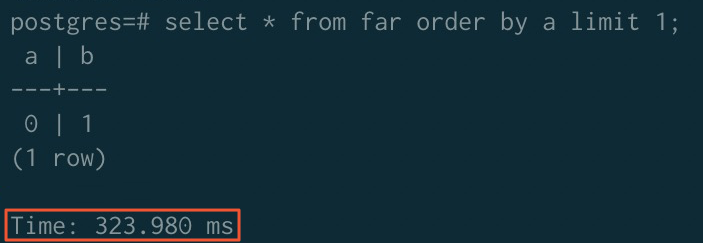• After sorting acceleration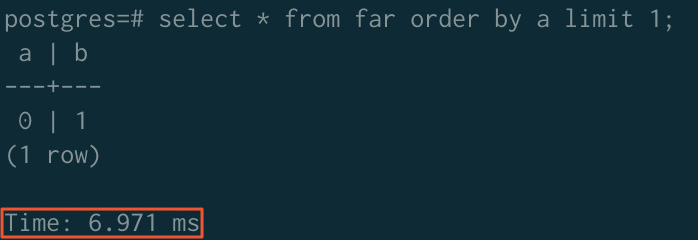• GROUP BY sorting acceleration
• Before sorting acceleration• After sorting acceleration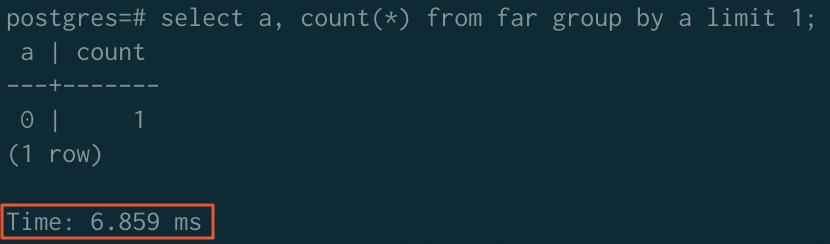• JOIN sorting acceleration
• Before sorting acceleration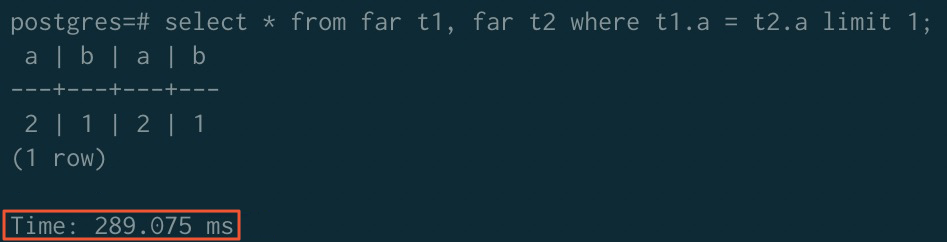• After sorting acceleration
Note If you want to use the sorting acceleration feature for JOIN operators, you must execute the following statements to disable the ORCA optimizer and enable the merge join algorithm:
``````SET enable_mergejoin TO on;
SET optimizer TO off;``````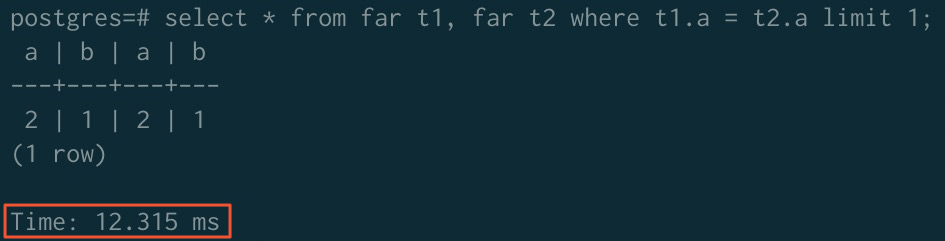- ORDER BY GROUP BY JOIN
Before acceleration 323.980 ms 779.368 ms 289.075 ms
After acceleration 6.971 ms 6.859 ms 12.315 ms